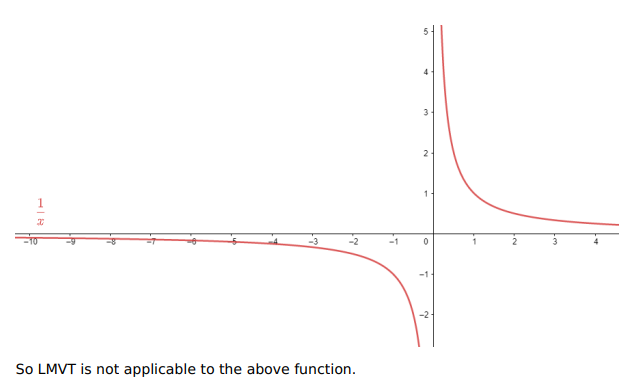# Show that Lagrange's mean-value theorem is not applicable

Question:

Show that Lagrange's mean-value theorem is not applicable to $f(\mathrm{x})=\frac{1}{\mathrm{x}}$ on $[-1,1]$

Solution:

Given:

Since the graph is discontinuous at $x=0$ as shown in the graph.